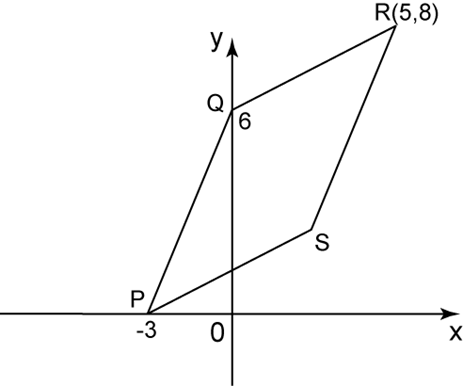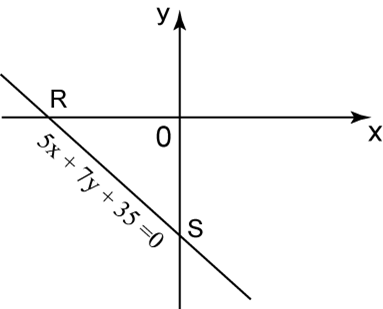# 5.7.2 The Straight Line, SPM Paper 2

Question 4:In the diagram above, PQRS is a parallelogram. Find
(b) the equation of QR,
(c)  the x-intercept of QR.

Solution:
(a)
PQ is parallel to SR, gradient of PQ = gradient of SR.

(b)

(c)
$\begin{array}{l}\text{For x-intercept,}y=0\\ 0=\frac{2}{5}x+6\\ x=-15\end{array}$
Therefore x-intercept of QR = –15.

Question 5:In the diagram above, a straight line 5x + 7y + 35 = 0 intersects with the x-axis and y-axis at R and S respectively. Determine
(a) the gradient of the straight line RS.
(b) the x-intercept of the straight line RS.
(c) the distance of RS.

Solution:
(a)
$\begin{array}{l}5x+7y+35=0\\ 7y=-5x-35\\ y=-\frac{5}{7}x-5\\ \therefore \text{The gradient of the straight line}RS=-\frac{5}{7}.\end{array}$

(b)
$\begin{array}{l}\text{At x-intercept,}y=0\\ 0=-\frac{5}{7}x-5\\ \frac{5}{7}x=-5\\ x=-7\\ \therefore \text{x-intercept of the straight line}RS=-7.\end{array}$

(c)
$\begin{array}{l}\text{Point}R=\left(-7,0\right)\text{and point}S=\left(0,-5\right)\\ \text{Distance of}RS=\sqrt{{\left(-7-0\right)}^{2}+{\left(0-\left(-5\right)\right)}^{2}}\\ \text{Distance of}RS=\sqrt{49+25}\\ \text{Distance of}RS=\sqrt{74}\text{units}\end{array}$

Question 6:In the diagram above, O is the origin of the Cartesian plane, AOB is a straight line and OA = AC. Find
(a) the coordinates of C.
(b) the value of h.
(c) the equation of BC.

Solution:
(a)
x-coordinate of C = –3 × 2 = –6
Therefore coordinates of C = (–6, 0).

(b)

(c)

### 1 thought on “5.7.2 The Straight Line, SPM Paper 2”

1.thank you for your exercise . it was good revision for my exam tommorow